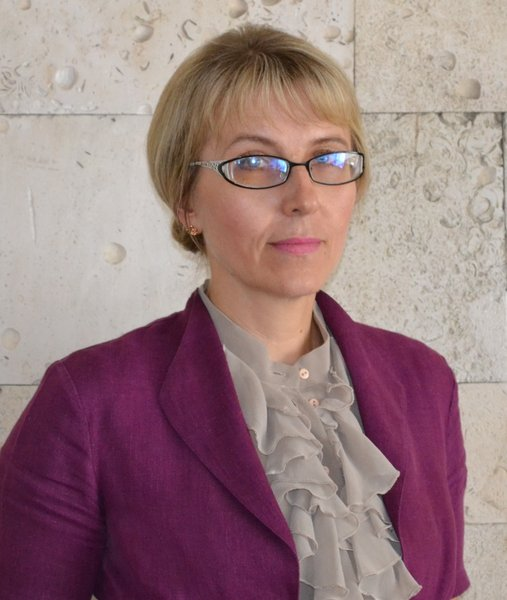Olena F. Kashpur
Deputy Dean for Educational and Methodical Work

### Information

 Position: Deputy Dean for Educational and Methodical Work Candidate of Physical and Mathematical Science Associate Professor 213 259-04-36 olena.kashpur@gmail.com approximation of nonlinear operators, spectral theory of operators, partial differential equations

Laureate of Prize of National Academy of Science for young scientist, 1996

### Courses:

Numerical methods
Numerical methods in informatics
Equations of mathematical physics
Numerical methods in mathematical physics

### Publications:

1. Integral Newton-Type Polynomials with Continual Nodes // Ukrainian Mathematical Journal. - June, 2003. - Vol. 55, № 6. - Р. 942 - 955.
2. Analysis of the Accuracy of Interpolation of Entire Operators in a Hilbert Space in the Case of Perturbed Nodal Values. // Ukrainian Mathematical Journal. - July, 2003. - Vol. 55, № 6. - P. 1153 – 1162.
3. On continual interpolation nodes for operators in linear topological spaces // Ukrainian Mathematical Journal. - Novermber, 2010. - Vol. 62, Iss.04. - P. 564 - 574.
4. До деяких питань поліноміальної інтерполяції в евклідових просторах. // Доповіді Національної академії наук України. - 2016. - № 10. - С. 10 – 14.
5. Invariance and uniqueness of solutions to polynomial interpolation problems in Euclidean space. // Journal of computational and applied mathematics. - 2015. - № 2. - P. 8 - 14.
6. Lagrange interpolation formula in linear spaces. // J. Comput. Math. – 2018. - № 2 (128). – P. 61- 67.
7. Інтерполяційний поліном Лагранжа в лінійному просторі зі скалярним добутком. // Доповіді Національної академії наук України. - 2018. - № 8. - С. 12 – 17.
8. Operator Interpolation and Systems of Linear Equations and Inequalities in Euclidean Spaces. / V. L. Makarov, V. V. Khlobystov, O. F. Kashpur // Ukrainian Mathematical Journal. - april, 2021. – Vol. 72. - № 11. - P. 1758 – 1770.
9. Hermite–Birkhoff Interpolation Polynomial of Minimum Norm in Hilbert Space // Cybernetics and Systems Analysis. - 2021. - Vol. 57, Iss. 5. - P. 803 – 808.
10. Hermite Interpolation Polynomial for Functions of Several Variables // Cybernetics and Systems Analysis. - 2022. - Vol. 58, Iss. 3. - P. 399 – 408.
11. Кашпур О.Ф., Макаров В.Л., Хлобистов В.В. До питання континуальності інтерполяційних вузлів для операторів у лінійних топологічних просторах. // Український математичний журнал. – 2010, – т. 62, № 4, – с. 494-503.
12. Кашпур О.Ф., Москальков М.М., Риженко А.І., Кузьмін А.В., Хлобистов В.В. Практикум з методів обчислень. Наближення функцій. Частина 2. // Київ, Вид-во МАУП, – 2008, 110 с.
13. Кашпур О.Ф., Малишева Т.М., Хлобистов В.В. Про точні на поліномах інтерполяційні формули в гільбертовому просторі. // Вісник Київського університету. Сер. фіз.-мат. науки, – 2007, – вип. 1, – с. 168-172.